Courses

# Test: Memory Management & Virtual Memory- 2

## 15 Questions MCQ Test Question Bank for GATE Computer Science Engineering | Test: Memory Management & Virtual Memory- 2

Description
This mock test of Test: Memory Management & Virtual Memory- 2 for Computer Science Engineering (CSE) helps you for every Computer Science Engineering (CSE) entrance exam. This contains 15 Multiple Choice Questions for Computer Science Engineering (CSE) Test: Memory Management & Virtual Memory- 2 (mcq) to study with solutions a complete question bank. The solved questions answers in this Test: Memory Management & Virtual Memory- 2 quiz give you a good mix of easy questions and tough questions. Computer Science Engineering (CSE) students definitely take this Test: Memory Management & Virtual Memory- 2 exercise for a better result in the exam. You can find other Test: Memory Management & Virtual Memory- 2 extra questions, long questions & short questions for Computer Science Engineering (CSE) on EduRev as well by searching above.
QUESTION: 1

### If the property of locality of reference is well pronounced a program: 1. The number of page faults will be more. 2. The number of page faults will be less. 3. The number of page faults will remain the same. 4. Execution will be faster.

Solution:

If the property of locality of reference is well pronounced then the page to be accessed will be found in the memory more likely and hence page faults will be less. Also since access time will be only of the upper level in the memory hierarchy bence execution will be faster.

QUESTION: 2

### Memory protection is of no use in a

Solution:

Even in a non-multiprogramming system, memory protection may be used, for example, spooling is being used.

QUESTION: 3

### In a paged memory, the page hit ratio is 0.35. The time required to access a page in secondary memory is equal to 100 ns. The time required to access a page in primary memory is 10 ns. The average time required to access a page is

Solution:

Hit ratio = 0.35
Time (secondary memory) = 100 ns
T(main memory) = 10 ns
Average access time = h(Tm) + (1 - h) (Ts)
= 0.35 x 10 +(0.65) x 100
= 3.5 + 65
= 68.5 ns

QUESTION: 4

The size of the virtual memory depends on the size of the

Solution:

The size of virtual memory depends on the size of the address bus. Processor generates the memory address as per the size of virtual memory.

QUESTION: 5

Solution:

In doing this user programs do not need any support from the O.S. or the hardware.

QUESTION: 6

Which one of the following is true?

Solution:
QUESTION: 7

Which of the following is true?

Solution:

By using the overlays we can execute much greater processes simultaneously which cannot be execute and reside in the memory at the same time. In this the process to be executed process brought to memory only when it is needed at the time of execution.

QUESTION: 8

Consider a computer with 8 Mbytes of main memory and a 128 K cache. The cache block size is 4 K. It uses a direct mapping scheme for cache management. How many different main memory blocks can map onto a given physical cache block?

Solution:

Number of block in cash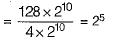Number of block in main memory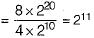Since the system uses direct mapping hence different memory blocks that can map into the cache block.
= Number of blocks in MM% Number of blocks in cache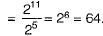QUESTION: 9

Consider a system using paging and segmentation. The virtual address space consist of up to 8 segments and each segment is 229 bytes long. The hardware pages each segment into 28 byte pages.

How many bits in the virtual address specify the offset within page?

Solution:

Virtual address space = 8 x 229 bytes
= 232 bytes
No. of pages within each segment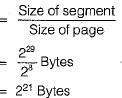Offset within the page = 8 bits (for 28 bytes)

QUESTION: 10

Consider a system using paging and segmentation. The virtual address space consist of up to 8 segments and each segment is 229 bytes long. The hardware pages each segment into 28 byte pages.

How many bits in the virtual address specify the entire virtual address?

Solution: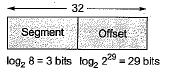QUESTION: 11

For 64 bit virtual addresses, a 4 KB page size and 256 MB of RAM, an inverted page table requires

Solution:

Virtul address space = 264 bytes
Page size = 4 KB
= 212 Bytes
Physical Memotry = 256 MB.
= 228 Bytes
Number of entries in inverted page table = Number of frames in physical memory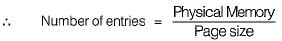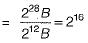QUESTION: 12

If page fault service time is 50 milliseconds and memory access time is 100 nanoseconds, then what will be the effective access time, if the probability of page fault is p.

Solution:

[(1- p)100 + p x 50 x 106] ns
Since, 1 ns = 10-9sec
=> 10-9[(1 -p)100 + p x 50 x 106] sec
=> [(1 - p) x 10-7 + p x 50 x 10-3] sec
=> 10-7 - 10-7p + p x 50 x 10-3
=> 10-7 + l0-3 x p f [50 - 10-4]
~ [10-7 + p x 10-3 x 49.9] sec

QUESTION: 13

Consider a system with 1 K pages and 512 frames and each page is of size 2 KB. How many bits are required to represent the virtual address space Memory is

Solution:

Virtual address space consists of pages.
Given that,
Number of pages = 1 K = 210
Page size = 2KB = 211B
= 211 x210
= 221 B
∴  21 bits are required to represent the virtual address space.

QUESTION: 14

Consider a virtual memory of 256 terabytes. The page size is 4K. This logical space is mapped into a physical memory of 256 megabytes.

How many bits are there in the virtual memory?

Solution:

Virtual address space = 256 terabytes
= 256 x 240 bytes
= 248 bytes [1 terabyte = 240 bytes] ∴ Number of bits to represent the virtual memory
= 48 bits

QUESTION: 15

Consider a virtual memory of 256 terabytes. The page size is 4K. This logical space is mapped into a physical memory of 256 megabytes.

How many pages are there in the virtual memory?

Solution:

Page size = 4 K
= 212 Bytes
Number of pages in virtual memory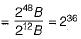=> 26.230 pages = 64 giga pages.